As a Go novice, I’m curious to see any “weird” code; for example, I recently saw a few methods; the pseudo-code is as follows.

 ``````1 2 3 4 5 6 7 8 `````` ``````func FindA() ([]*T,error) { } func FindB() ([]T,error) { } func SaveA(data *[]T) error { } func SaveB(data *[]*T) error { } ``````

I believe that most newcomers to `Go` are confused when they see this code, the most confusing of which is

 ``````1 2 3 `````` ``````[]*T *[]T *[]*T ``````

This declaration of a slice, without looking at the next two ways of writing it, is quite understandable when looking at `[]*T` alone. The slice holds the memory addresses of all T, which is more space-efficient than storing T itself, and `[]*T` can modify the value of T inside the method, while `[]T` cannot.

 `````` 1 2 3 4 5 6 7 8 9 10 11 12 13 14 15 16 17 18 `````` ``````func TestSaveSlice(t *testing.T) { a := []T{{Name: "1"}, {Name: "2"}} for _, t2 := range a { fmt.Println(t2) } _ = SaveB(a) for _, t2 := range a { fmt.Println(t2) } } func SaveB(data []T) error { t := data t.Name = "1233" return nil } type T struct { Name string } ``````

For example, the above example prints

 ``````1 2 3 4 `````` ``````{1} {2} {1} {2} ``````

Only by modifying the method to

 ``````1 2 3 4 5 `````` ``````func SaveB(data []*T) error { t := data t.Name = "1233" return nil } ``````

to modify the value of T:

 ``````1 2 3 4 `````` ``````&{1} &{2} &{1233} &{2} ``````

## Example

Let’s focus on the difference between `[]*T` and `*[]T`, where two `append` functions are written.

 ``````1 2 3 4 5 6 7 8 9 `````` ``````func TestAppendA(t *testing.T) { x:=[]int{1,2,3} appendA(x) fmt.Printf("main %v\n", x) } func appendA(x []int) { x= 100 fmt.Printf("appendA %v\n", x) } ``````

Looking at the first one first, the output is the result of

 ``````1 2 `````` ``````appendA [1000 2 3] main [1000 2 3] ``````

It shows that the modifications inside the function can affect the outside during function passing.

Here we look at another example.

 ``````1 2 3 4 `````` ``````func appendB(x []int) { x = append(x, 4) fmt.Printf("appendA %v\n", x) } ``````

The end result is

 ``````1 2 `````` ``````appendA [1 2 3 4] main [1 2 3] ``````

There is no impact to the outside.

And when we adjust it a bit more, we find that it is different again.

 ``````1 2 3 4 5 6 7 8 9 `````` ``````func TestAppendC(t *testing.T) { x:=[]int{1,2,3} appendC(&x) fmt.Printf("main %v\n", x) } func appendC(x *[]int) { *x = append(*x, 4) fmt.Printf("appendA %v\n", x) } ``````

End result.

 ``````1 2 `````` ``````appendA &[1 2 3 4] main [1 2 3 4] ``````

You can see that if you pass a pointer to a slice, using the `append` function to append data will affect the outside.

## slice Principle

Before analyzing the above three cases, let’s understand the data structure of `slice`.

A direct look at the source code shows that slice is actually a structure, but it is not directly accessible to the public.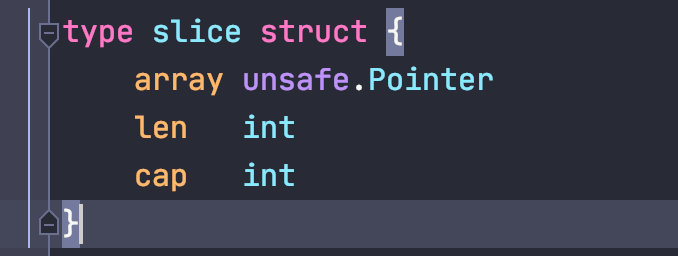Source code address `runtime/slice.go`

There are three important attributes.

attribute Meaning
array The array that holds the data at the bottom is a pointer.
len Slice length
cap Slicing capacity cap>=len

When it comes to slicing, one has to think of arrays, which can be understood as follows.

Slicing is an abstraction of arrays, and arrays are the underlying implementation of slicing.

In fact, it is easy to see by the name slicing that it is cutting a part of the array; as opposed to the fixed size of the array, slicing can be expanded according to the actual usage.

So a slice can also be obtained by “slicing” the array.

 ``````1 2 3 `````` ``````x1:=int{0,1,2,3,4,5} x2 := x[1:4] fmt.Println(len(x2), cap(x2)) ``````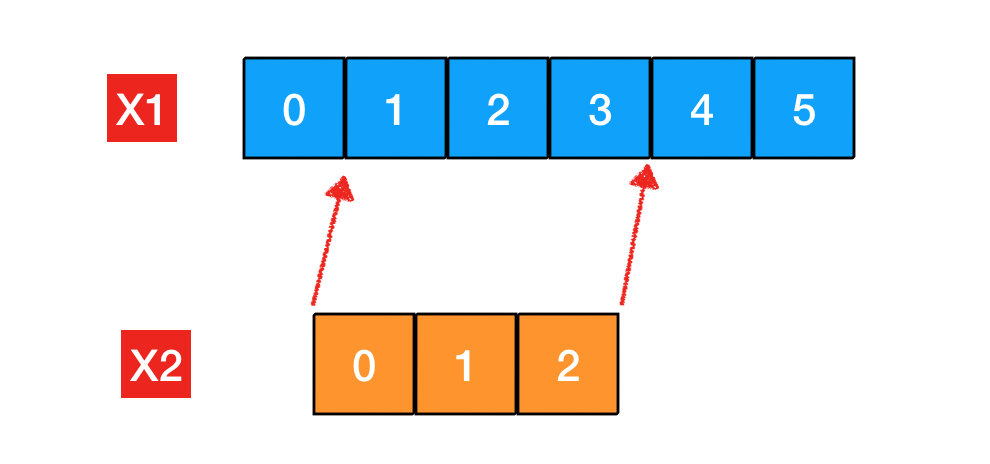where the length and capacity of `x1` are 6.

The length and capacity of `x2` are 3 and 5.

• The length of * x2 is easy to understand.
• The capacity is equal to 5, which is the maximum length that can be used for the current slice.

Since slice x2 is a reference to array x1, the underlying array excluding the left position that is not referenced is the maximum capacity of the slice, which is 5.

### The same underlying array

Take the code just shown as an example.

 ``````1 2 3 4 5 6 7 8 9 `````` ``````func TestAppendA(t *testing.T) { x:=[]int{1,2,3} appendA(x) fmt.Printf("main %v\n", x) } func appendA(x []int) { x= 100 fmt.Printf("appendA %v\n", x) } ``````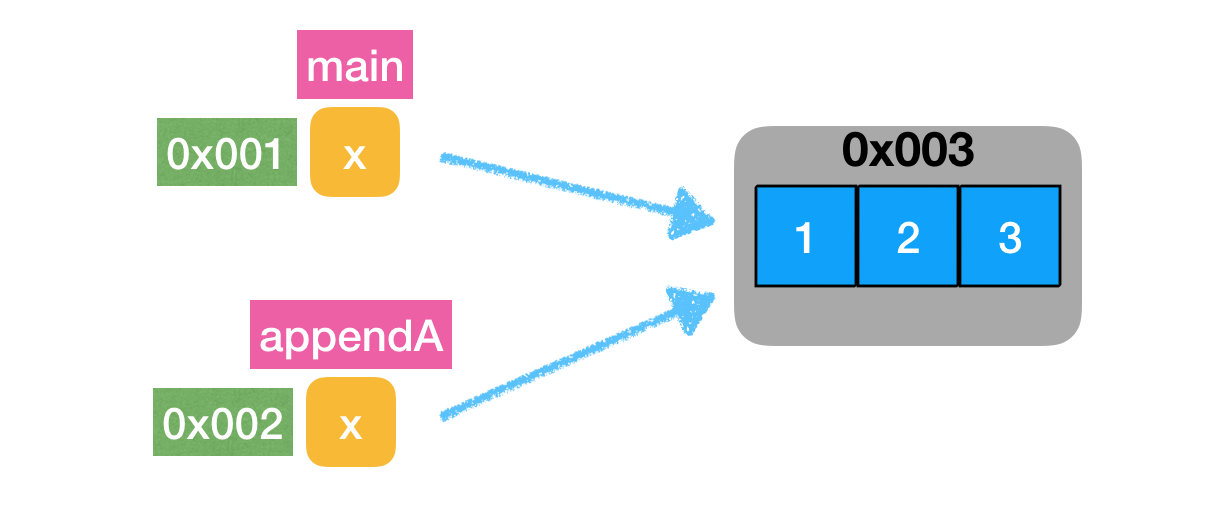During function passing, the x in `main` is referenced by the same array as the x slice in the `appendA` function.

So for `x=100` in the function, the `main` function can also get it.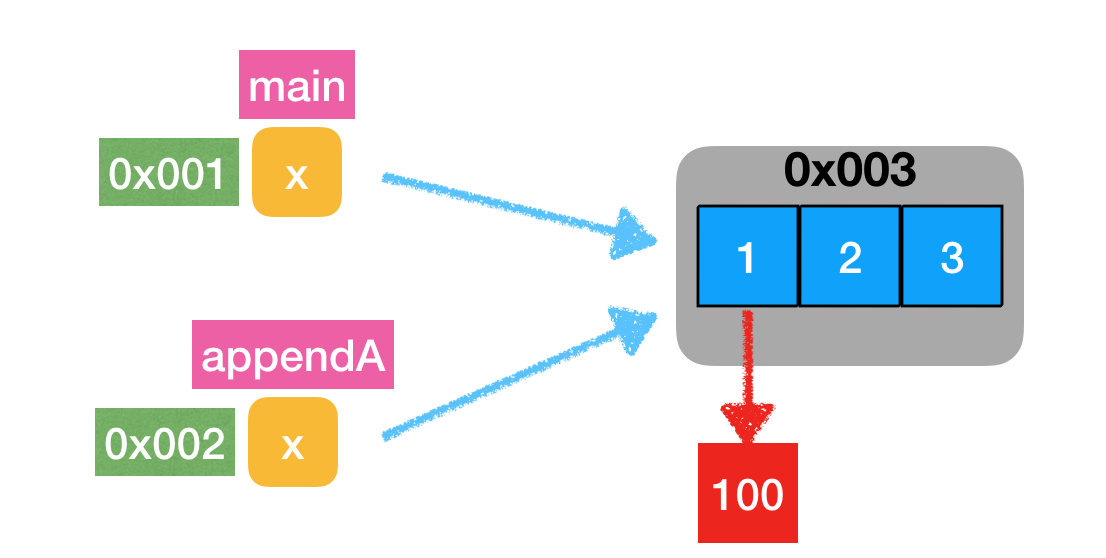Essentially the same block of memory data is being modified.

### Misunderstandings caused by value passing

In the above example, after calling the `append` function in `appendB` to append data, you will find that the main function is not affected, here I have slightly adjusted the sample code.

 `````` 1 2 3 4 5 6 7 8 9 10 11 12 13 `````` ``````func TestAppendB(t *testing.T) { //x:=[]int{1,2,3} x := make([]int, 3,5) x = 1 x = 2 x = 3 appendB(x) fmt.Printf("main %v len=%v,cap=%v\n", x,len(x),cap(x)) } func appendB(x []int) { x = append(x, 444) fmt.Printf("appendB %v len=%v,cap=%v\n", x,len(x),cap(x)) } ``````

The main reason is the modification of the slice initialization method, so that the capacity is greater than the length, the specific reason will be explained subsequently.

The output is as follows.

 ``````1 2 `````` ``````appendB [1 2 3 444] len=4,cap=5 main [1 2 3] len=3,cap=5 ``````

The data in the `main` function does seem to be unaffected; however, those who are careful will notice that the length of x in the `appendB` function changes from +1 to 4 after `append()`.

In the `main` function, the length is changed back to 3.

This difference in detail is why `append()` “seems” not to work; as to why it “seems”, the code has been adjusted again to

 `````` 1 2 3 4 5 6 7 8 9 10 11 `````` ``````func TestAppendB(t *testing.T) { //x:=[]int{1,2,3} x := make([]int, 3,5) x = 1 x = 2 x = 3 appendB(x) fmt.Printf("main %v len=%v,cap=%v\n", x,len(x),cap(x)) y:=x[0:cap(x)] fmt.Printf("y %v len=%v,cap=%v\n", y,len(y),cap(y)) } ``````

On top of that, a slice is made based on x after `append`; the scope of the slice is all the data in the array referenced by x.

Let’s see what happens.

 ``````1 2 3 `````` ``````appendB [1 2 3 444] len=4,cap=5 main [1 2 3] len=3,cap=5 y [1 2 3 444 0] len=5,cap=5 ``````

You will magically find that y prints out all the data, and the data appended in the `appendB` function has actually been written to the array, but why doesn’t `x` itself get it?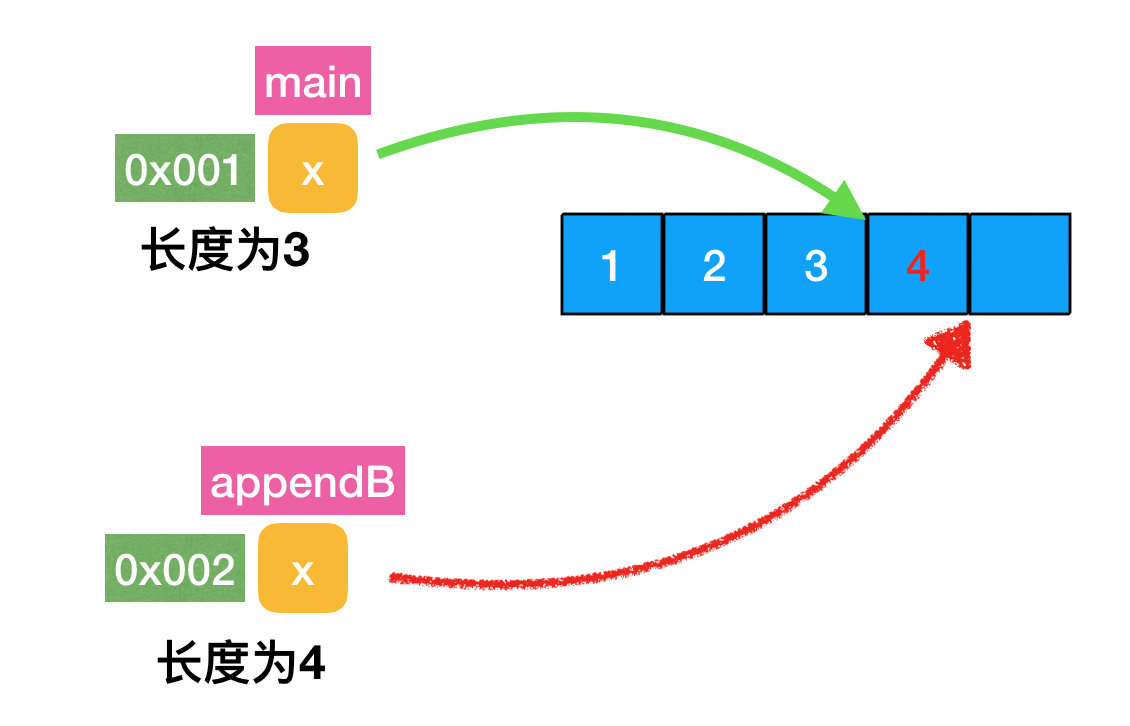It is easy to understand by looking at the figure.

• It is true that in `appendB` the data is appended to the original array and the length is also increased.
• But since it is a value pass, the structure `slice`, even if it changes the length to 4, only changes the length of the copied object, and the length in `main` is still 3.
• Since the underlying array is the same, we can see the additional data by regenerating a full-length slice based on this underlying array.

So the essential reason here is that `slice` is a structure, which is passed as a value, and no matter how the length is modified in the method, it does not affect the original data (in this case, the length and capacity properties).

### Expansion of slices

One more note.

It was specifically mentioned earlier that the example here is slightly altered, mainly by setting the capacity of the slice to exceed the length of the array.

What happens if this special setting is not made?

 `````` 1 2 3 4 5 6 7 8 9 10 11 12 13 14 15 `````` ``````func TestAppendB(t *testing.T) { x:=[]int{1,2,3} //x := make([]int, 3,5) x = 1 x = 2 x = 3 appendB(x) fmt.Printf("main %v len=%v,cap=%v\n", x,len(x),cap(x)) y:=x[0:cap(x)] fmt.Printf("y %v len=%v,cap=%v\n", y,len(y),cap(y)) } func appendB(x []int) { x = append(x, 444) fmt.Printf("appendB %v len=%v,cap=%v\n", x,len(x),cap(x)) } ``````

Output results.

 ``````1 2 3 `````` ``````appendB [1 2 3 444] len=4,cap=6 main [1 2 3] len=3,cap=3 y [1 2 3] len=3,cap=3 ``````

At this point, you will find that the y slice data in the main function has not changed either, why is this?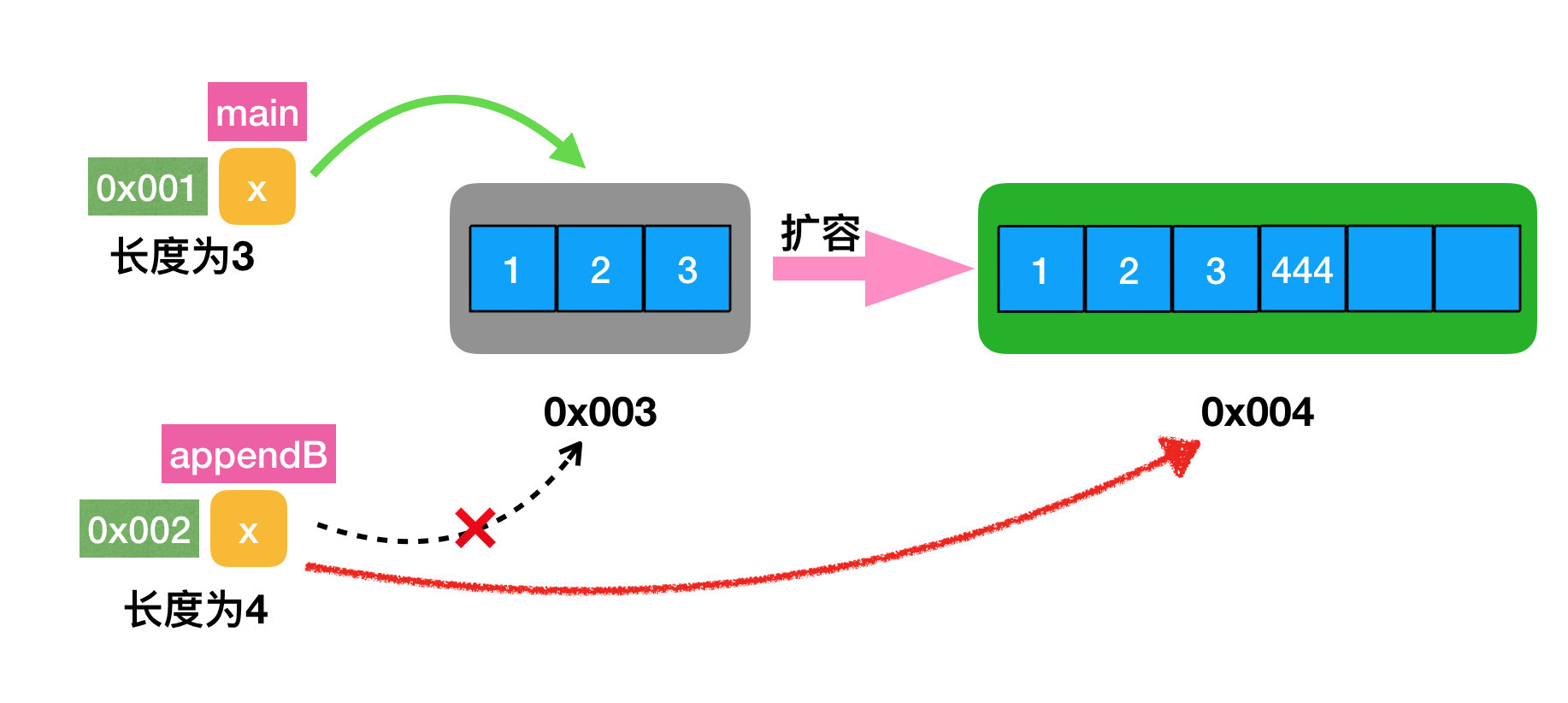This is because the length and capacity of the initialized x slice is 3. When appending data in the `appendB` function, we find that there is no more room.

This is when the expansion is done.

• Copy a copy of the old data into the new array.
• Append the data.
• Return the new data memory address to x in `appendB` .

Again, since this is value passing, slicing the underlying array in `appendB` has no effect on the slices in the `main` function, which results in no change to the data in the final `main` function.

### Passing a slice pointer

Is there any way to have an external impact even during expansion?

 ``````1 2 3 4 5 6 7 8 9 `````` ``````func TestAppendC(t *testing.T) { x:=[]int{1,2,3} appendC(&x) fmt.Printf("main %v len=%v,cap=%v\n", x,len(x),cap(x)) } func appendC(x *[]int) { *x = append(*x, 4) fmt.Printf("appendC %v\n", x) } ``````

The output results are.

 ``````1 2 `````` ``````appendC &[1 2 3 4] main [1 2 3 4] len=4,cap=6 ``````

This is when external slices can be affected, and the reason is actually quite simple; the

As I said earlier, since `slice` itself is a structure, when we pass a pointer, it’s the same principle as the usual custom `struct` that modifies data inside a function via a pointer.

In the end, the pointer to x in `appendC` points to the expanded structure, and since the pointer to x in the main function is passed, the same x in the main function also points to the structure.

## Summary

So to summarize.

• A slice is an abstraction of an array, while a slice is itself a structure.
• The same array is referenced inside and outside the function when parameters are passed, so changes to the slice will affect the outside of the function.
• The situation will change if expansion occurs, and also expansion will lead to data copying; so try to anticipate the slice size to avoid data copying.
• When regenerating slices for slices or arrays, the data will affect each other because the same underlying array is shared, and this needs to be noted.
• Slices can also pass pointers, but the scenario is rare and will bring unnecessary misunderstandings; it is recommended that values be passed to values, as length and capacity do not take up much memory.

I believe that the use of slices will find very similar to `Java` in the `ArrayList`, the same is based on array implementation, will also be expanded to occur data copy; so it seems that the language is only the choice of the upper layer use, some common underlying implementation of everyone is similar.

At this point we then look at the title `[]*T *[]T *[]*T` will find that there is no connection between these, just look like easy to bluff.

Reference `https://crossoverjie.top/2021/07/28/go/slice%20pointer/`